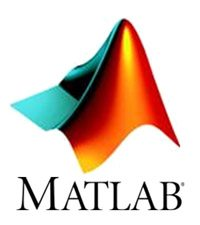Atithya Amaresh1. Numerical Computing with Matlab

Author: by Cleve B. Moler
Publisher: SIAM, 2004

This textbook provides an introductory course in numerical methods, MATLAB, and technical computing. It emphasis on explaining the use of mathematical software. The book has used computer graphics, including interactive graphical expositions of numerical algorithms. The topics include introduction to MATLAB; linear equations; interpolation; zeros and roots; least squares; quadrature; ordinary differential equations; Fourier analysis; random numbers; eigenvalues and singular values; and partial differential equations.

2. Experiments with MATLAB

Author: Cleve B. Moler
Publisher: SIAM, 2008

The book introduces Matlab by way of examples and experiments. A lot of experiments involve understanding and modifying Matlab scripts and functions. A reader should have access to Matlab and to SIAM’s exm toolbox, the collection of programs and data that are described in Experiments with MATLAB.

Author: Allen Downey
Publisher: Green Tea Press, 2008

An introductory textbook for people who have not programmed before. Covers basic MATLAB programming with emphasis on modeling and simulation of physical systems. The book starts with scalar values and works up to vectors and matrices very gradually.

4. MATLAB Programming

Publisher: Wikibooks, 2012

MATLAB is a numerical computing environment and fourth-generation programming language developed by MathWorks. It started out as a matrix programming language where linear algebra programming was simple. It can be run both under interactive sessions and as a batch job.

5. Digital Signal Processing Using MATLAB for Students and Researchers

Author: John W. Leis
Publisher: Wiley

With its active, hands-on learning approach, this text enables readers to master the underlying principles of digital signal processing and its many applications in industries such as digital television, mobile and broadband communications, and medical/scientific devices. Carefully developed MATLAB examples throughout the text illustrate the mathematical concepts and use of digital signal processing algorithms. Readers will develop a deeper understanding of how to apply the algorithms by manipulating the codes in the examples to see their effect. Moreover, plenty of exercises help to put knowledge into practice solving real-world signal processing challenges.